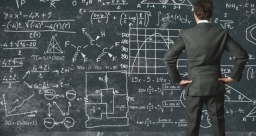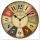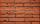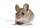# Six te 2

If 3t-7=5t, then 6t=

x =  -21

### Step-by-step explanation:Did you find an error or inaccuracy? Feel free to write us. Thank you!Tips to related online calculators
Do you have a linear equation or system of equations and looking for its solution? Or do you have a quadratic equation?

## Related math problems and questions:

• If-then equationIf 5x - 17 = -x + 7, then x =
• Eq1Solve equation: 4(a-3)=3(2a-5)
• Expression 1What is 7+8-(5×2)+5-4+(6×(5-3)+6)-(8+10)-7+6?
• Expression plus minusEvaluate expression: (-1)2 . 12 – 6 : 3 + (-3) . (-2) + 22 – (-3) . 2
• Evaluate 11Multiply the quotient of 6 and 2 by 3, then add 1. Add 1 and 6, then divide by 2 and multiply by 3. Divide 6 by the product of 2 and 3, then add 1.
• Temperature variationsToday's temperature was 80 degrees, and then the temperature dropped 10 degrees. Then it dropped 15 degrees again, then the next day, the temperature went up 2 degrees. What would the temperature be?
• MinutesDetermine the difference in minutes: T1 = 2 3/20 h T2 = 2.3 h
• Wood 11Father has 12 1/5 meters long wood. Then I cut the wood into two pieces. One part is 7 3/5 meters long. Calculate the length of the other wood?
• Evaluate expressionIf x=2, y=-5 and z=3 what is the value of x-2y
• FractionsSort fractions z1 = (6)/(11); z2 = (10)/(21); z3 = (19)/(22) by its size. Result write as three serial numbers 1,2,3.
• Temperature 2Sunday's high temperature was 3 degrees higher than Saturday's. On Monday, the temperature fell 5 degrees, then rose 7 degrees on Tuesday and 4 more on Wednesday. Then it fell 17 degrees to a record low of 31 on Thursday. What was the temperature on Satur
• Intersect of setsRefer to the sets below: U = {1,2,3,. .. ,10} A = {1,2,3,4,5} B = {2,4,6,7,8} C = {4,5,6,8,10} A ∩ B ∩ C = ___Why does 1 3/4 + 2 9/10 equal 4.65? How do you solve this?3/4 bricks weighs 6 kg and 2/3 bricks. How weighs one whole brick?3 cats eat 3 mice in 3 days. How many mice will be eaten by 10 cats in 10 days?Add two mixed fractions: 2 4/6 + 1 3/6The thermometer showed -12 degrees Celsius in the morning then the temperature rises by 4 degrees and later again increased by 2 degrees at the evening has fallen by 5 degrees and then falls 3 degrees. What end temperature does the thermometer show?